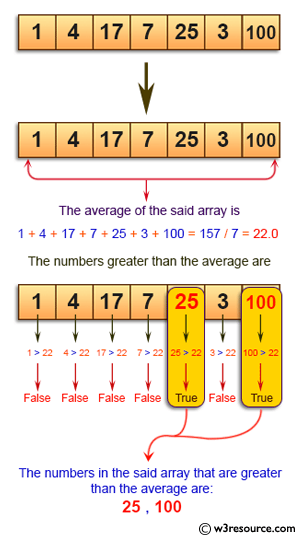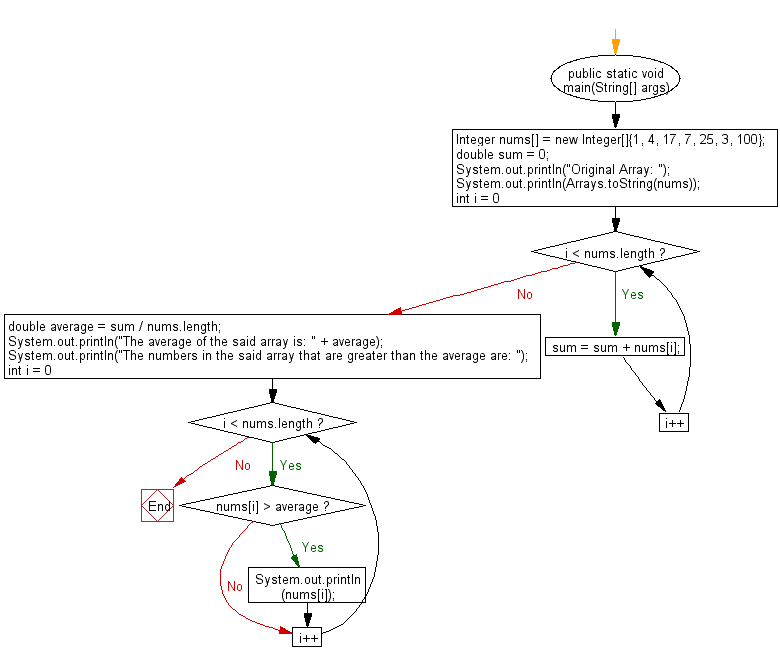﻿ Java programming exercises: Find the numbers greater than the average of the numbers of a specified array - w3resource# Java Exercises: Find the numbers greater than the average of the numbers of a specified array

## Java Basic: Exercise-162 with Solution

Write a Java program to find the numbers greater than the average of the numbers of a given array.

Pictorial Presentation:Sample Solution:

Java Code:

``````import java.util.*;
public class Solution {
public static void main(String[] args)
{
Integer nums[] = new Integer[]{1, 4, 17, 7, 25, 3, 100};
int sum = 0;
System.out.println("Original Array: ");
System.out.println(Arrays.toString(nums));
for(int i = 0; i < nums.length; i++) {
sum = sum + nums[i];
}
double average = sum / nums.length;
System.out.println("The average of the said array is: " + average);
System.out.println("The numbers in the said array that are greater than the average are: ");
for(int i = 0; i < nums.length; i++) {
if(nums[i] > average) {
System.out.println(nums[i]);
}
}
}
}
```
```

Sample Output:

```Original Array:
[1, 4, 17, 7, 25, 3, 100]
The average of the said array is: 22.0
The numbers in the said array that are greater than the average are:
25
100
```

Flowchart:Java Code Editor:

What is the difficulty level of this exercise?

﻿

## Java: Tips of the Day

Array vs ArrayLists:

The main difference between these two is that an Array is of fixed size so once you have created an Array you cannot change it but the ArrayList is not of fixed size. You can create instances of ArrayLists without specifying its size. So if you create such instances of an ArrayList without specifying its size Java will create an instance of an ArrayList of default size.

Once an ArrayList is full it re-sizes itself. In fact, an ArrayList is internally supported by an array. So when an ArrayList is resized it will slow down its performance a bit as the contents of the old Array must be copied to a new Array.

At the same time, it's compulsory to specify the size of an Array directly or indirectly while creating it. And also Arrays can store both primitives and objects while ArrayLists only can store objects.

Ref: https://bit.ly/3o8L2KH